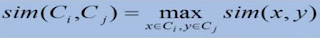# New Technology

Trending Technology Machine Learning, Artificial Intelligent, Block Chain, IoT, DevOps, Data Science## Search This Blog

Hierarchical Clustering

• Produce a nested sequence of clusters.
• One approach : recursive application of a partitional clustering algorithm.
Types of hierarchical clustering

Agglomerative (bottom up) clustering : builds the dendrogram (tree) from the bottom level, and
• merges the most similar (or nearest) pair of clusters
• stops when all the data points are merged into a single cluster (i.e., the root cluster).
Divisive (top down ) clustering : It starts with all points in one cluster, the root.
• Splits the root into a set of child clusters. Each child cluster is recursively divided further
• stops when only singleton clusters of individual data points remain, i.e., each cluster with only a single point
Dendrogram : Hierarchical ClusteringDendrogram
• Given an input set S
• nodes represent subsets of S
• Features of the tree :
• The root is the whole input set S.
• The leaves are the individual elements of S.
• The internal nodes are defined as the union of their children.

Dendrogram : Hierarchical ClusteringDendrogram
→ Each level of the tree represents a partition of the input data into several (nested) clusters or groups.
→ May be cut at any level : Each connected component forms a cluster.Hierrarchical Agglomerative clustering
• Initially each data point forms a cluster.
• Compute the distance matrix between the clusters.
• Repeat -
- Merge the two closest clusters
- Update the distance matrix
• Until only a single cluster remains.
Different definitions of the distance leads to different algorithms.

Initialization
• Each individual point is taken as a cluster
• Construct distance/proximity matrixIntermediate State
• After some merging steps , we have some clustersAfter Merging
• Update the distance matrixClosest Pair
• A few ways to measure distances of two clusters.
• Complete-link → Similarity of the least similar points
• Centroid → Clusters whose centroids (centers of gravity) are the most similar
• Average-link → Average cosine between pairs of elements

Distance between two clusters
• Single-link distance between clusters Ci and Cj is the minimum distance between any object in Ci and any object in Cj• Determined by one pair of points, i.e., by one link in the proximity graph.• The distance between two clusters is the distance of two furthest data points in the two clusters.

• Makes "tighter" spherical clusters that are typically preferable.
• It is sensitive to outliers because they are far away
Computational Complexity
• In the first iteration, all HAC methods need to compute similarity of all pairs of N initial instances, which is O(N2).
• In each of the subsequent N-2 merging iterations, compute the distance between the most recently created cluster and all other existing clusters.
• In order to maintain an overall O(N2) performance , computing similarity to each other cluster must be done in constant time.
• Often O(N3) if done naively or O(N2 log N) if done more cleverly